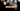# Leetcode | Solution of To Lower Case in JavaScript

March 29th, 2020
|

In this post, we will solve the problem to lower case from leetcode. Let's begin.

# Problem Statement

The question can be found at leetcode to lower case problem.

The problem states that we are given a string, and we need to convert it to lower case.

# Solution

One funny solution is to use the toLowerCase method. But that is the method we need to implement so we cannot use library methods.

The approach is as follows

• we will loop through each character in the string
• if the character is in upper case, we'll convert it to lower case else move on to the next character
• we will need to convert the characters to ASCII and back to string in the operation

We have discussed the approach, I urge you to go ahead on leetcode and give it another try.If you are here, it means something went wrong in implementation or you are just too lazy. In any case, let's see a simple implementation of the above logic.
``````const isUpperCase = char => {
let ascii = char.charCodeAt(0);

if (ascii >= 65 && ascii <= 90) {
return true;
}
return false
}

const toLower = char => {
let ascii = char.charCodeAt(0);

ascii = String.fromCharCode(ascii + 32);

return ascii
}

var toLowerCase = function (str) {
return str.split("")
.map(s => isUpperCase(s) ? toLower(s) : s)
.join("");
};``````

Let's look at the solution. We have three functions here

### isUpperCase

This function checks if the character in the argument is uppercase or not. We first convert the character into ASCII and check if the value is between `65 and 90` i.e. ASCII values of `'A' and 'Z'`.

### toLower

This function converts the given character into a lower case.

### toLowerCase

It is the function provided in the leetcode editor by default which we need to implement. First, we convert the string into a string array, then we map (loop over) the array of single string characters. Inside the map, we have a ternary operation which we use to check if the character is upper case or not. Then, we convert the character to lowercase or return it as it is respectively. At last, we join all the characters to form a string.

On submission, here are the stats

``````Status: Accepted
Runtime: 48ms
Memory: 34MB``````

## Time and space complexity

### Time complexity

We are using the map, split, join methods but they are chained as opposed to nested. So time complexity would be O(n).

### Space complexity

We are not using extra space except for some variable to store ASCII values and characters, thus, space complexity would be O(1).

# Summary

So, we solved the to lower case using some ASCII operations and finally, calculated the time and space complexities.

I hope you enjoyed solving this question. This is it for this one, complete source code for this post can be found on my Github Repo. Will see you in the next one.